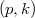﻿

### Inequalities concerning the$(p,k)$-gamma and$(p,k)$-polygamma functions

#### Abstract

In this paper, we establish several inequalities involving the$(p,k)$-gamma and$(p,k)$-polygamma functions. Among other tools, we employ the mean value therorem, the Hermite-Hadamard's inequality, Petrovic's inequality and the Holder's inequality. Upon some parameter variations, we recover some known results as special cases of the established results.

DOI Code: 10.1285/i15900932v38n2p93

Keywords: \$(p,k)\$-gamma function; \$(p,k)\$-digamma function; \$(p,k)\$-polygamma functions; inequality

Full Text: PDF